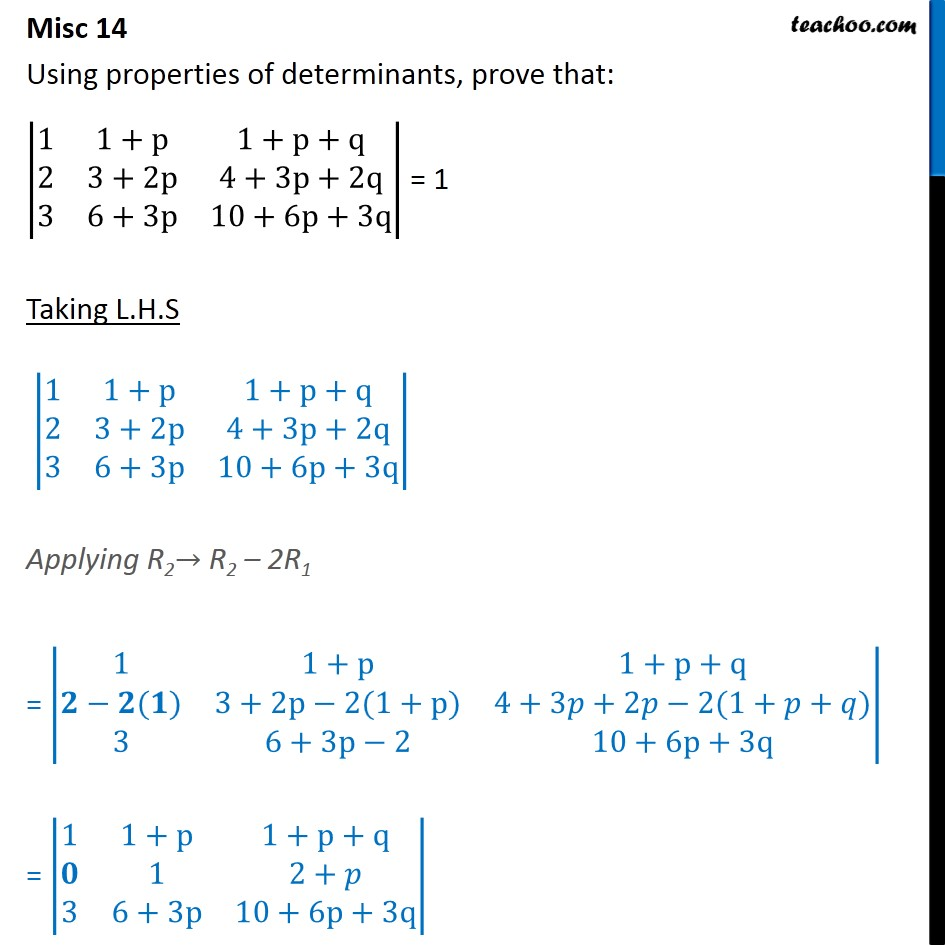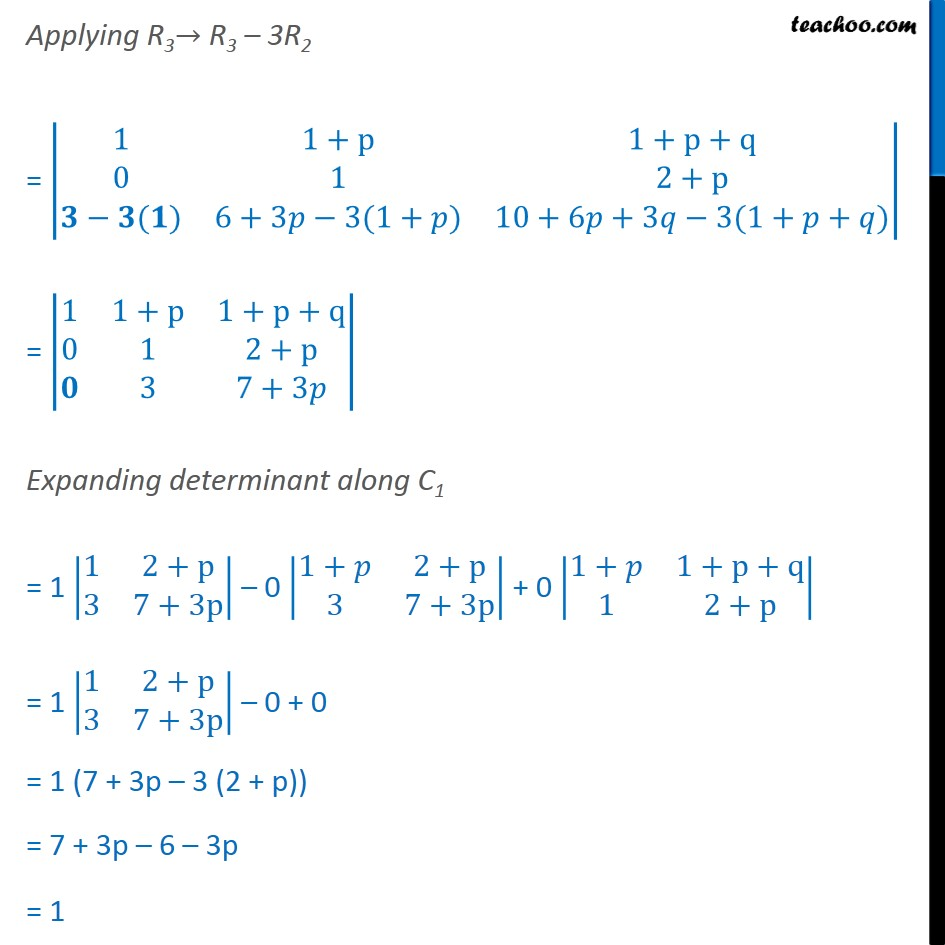Miscellaneous

Chapter 4 Class 12 Determinants (Term 1)
Serial order wise### Transcript

Misc 14 Using properties of determinants, prove that: 1﷮1+p﷮1+p+q﷮2﷮3+2p﷮4+3p+2q﷮3﷮6+3p﷮10+6p+3q﷯﷯ = 1 Taking L.H.S 1﷮1+p﷮1+p+q﷮2﷮3+2p﷮4+3p+2q﷮3﷮6+3p﷮10+6p+3q﷯﷯ Applying R2→ R2 – 2R1 = 1﷮1+p﷮1+p+q﷮𝟐−𝟐(𝟏)﷮3+2p−2(1+p)﷮4+3𝑝+2𝑝−2(1+𝑝+𝑞)﷮3﷮6+3p−2﷮10+6p+3q﷯﷯ = 1﷮1+p﷮1+p+q﷮𝟎﷮1﷮2+𝑝﷮3﷮6+3p﷮10+6p+3q﷯﷯ Applying R3→ R3 – 3R2 = 1﷮1+p﷮1+p+q﷮0﷮1﷮2+p﷮𝟑−𝟑(𝟏)﷮6+3𝑝−3(1+𝑝)﷮10+6𝑝+3𝑞−3(1+𝑝+𝑞)﷯﷯ = 1﷮1+p﷮1+p+q﷮0﷮1﷮2+p﷮𝟎﷮3﷮7+3𝑝﷯﷯ Expanding determinant along C1 = 1 1﷮2+p﷮3﷮7+3p﷯﷯ – 0 1+𝑝﷮2+p﷮3﷮7+3p﷯﷯ + 0 1+𝑝﷮1+p+q﷮1﷮2+p﷯﷯ = 1 1﷮2+p﷮3﷮7+3p﷯﷯ – 0 + 0 = 1 (7 + 3p – 3 (2 + p)) = 7 + 3p – 6 – 3p = 1 = R.H.S Hence Proved